# Advanced Turbo Decoding and Iterative Stopping Rule Appendix

NOTE: This section is Under-Edit if necessary: Construction began on September 20, 2015 and was finished on September 22, 2015. Update October 10, 2015.

Turbo Coded Signaling over a Coherent Memoryless Channel: Iterative Turbo Code Decoder and Cross-Entropy Stopping Rule Appendix

```by Darrell A. Nolta
September 22, 2015 with October 10, 2015 Update
```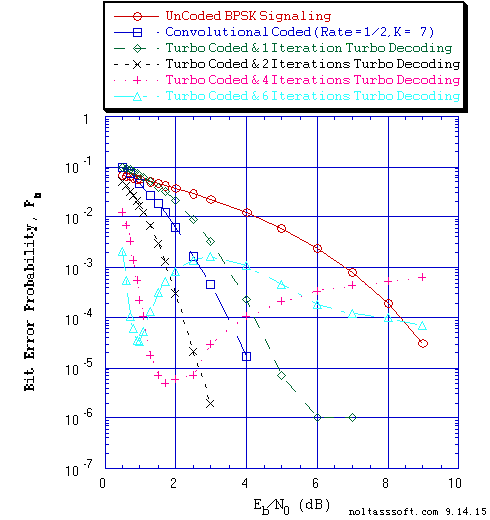```Figure 5. Bit Error Probability for UnCoded, Rate = 1/2 Convolutional Coded,
and Rate = 1/3 Turbo Coded (using a 6144-Bit QPP Interleaver) BPSK
Signaling over a Coherent Memoryless Channel with Additive White
Gaussian Noise (AWGN):
```
```          Equal probable Independent and Identical Distributed (IID) Source
for 10 Million, 1 Million, and 1,001,472 Information Bits for
UnCoded, Convolutional Coded, and Turbo Coded BPSK Signaling
respectively over a Vector Channel;
```
```          Rate = 1/2, Constraint Length K = 7 (3,1,0,3,3,2,3) a Best
(Optimal) Non-Recursive Convolutional Code (J.P. Odenwalder) and
Viterbi Algorithm using a Path Memory Length of 35 bits and an
Unquantized Branch Metric;
```
```          Rate (r) = 1/3, K = 3 Turbo Code based on a r = 1/2, K = 3 (G0 = 7
octal, G1 = 5 octal) Recursive Systematic Convolutional component
code and a 6144-Bit QPP Turbo Encoder Interleaver; and
```
```          Log-MAP Iterative Turbo Decoder using Systematic Channel Output as
input to both component decoders and Decode Information Bit Decision
is based on the Sum of both decoders' log-likelihood ratios
(L-values) and for One Iteration, Two Iterations, Four Iterations,
and Six Iterations Fixed Number Stopping Rule.
```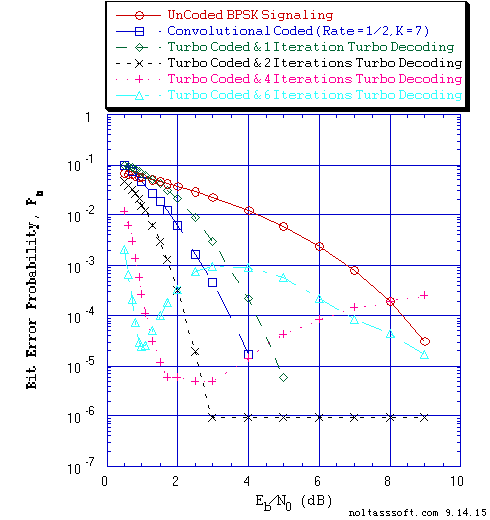```Figure 6. Bit Error Probability for UnCoded, Rate = 1/2 Convolutional Coded,
and Rate = 1/3 Turbo Coded (using a 32768-Bit QPP Interleaver) BPSK
Signaling over a Coherent Memoryless Channel with Additive White
Gaussian Noise (AWGN):
```
```          Equal probable Independent and Identical Distributed (IID) Source
for 10 Million, 1 Million, and 1,001,472 Information Bits for
UnCoded, Convolutional Coded, and Turbo Coded BPSK Signaling
respectively over a Vector Channel;
```
```          Rate = 1/2, Constraint Length K = 7 (3,1,0,3,3,2,3) a Best (Optimal)
Non-Recursive Convolutional Code (J.P. Odenwalder) and Viterbi
Algorithm using a Path Memory Length of 35 bits and an Unquantized
Branch Metric;
```
```          Rate (r) = 1/3, K = 3 Turbo Code based on a r = 1/2, K = 3 (G0 = 7
octal, G1 = 5 octal) Recursive Systematic Convolutional component
code and a 32768-Bit QPP Turbo Encoder Interleaver; and
```
```          Log-MAP Iterative Turbo Decoder using Systematic Channel Output as
input to both component decoders and Decode Information Bit
Decision is based on the Sum of both decoders' log-likelihood ratios
(L-values) and for One Iteration, Two Iterations, Four Iterations,
and Six Iterations Fixed Number Stopping Rule.
```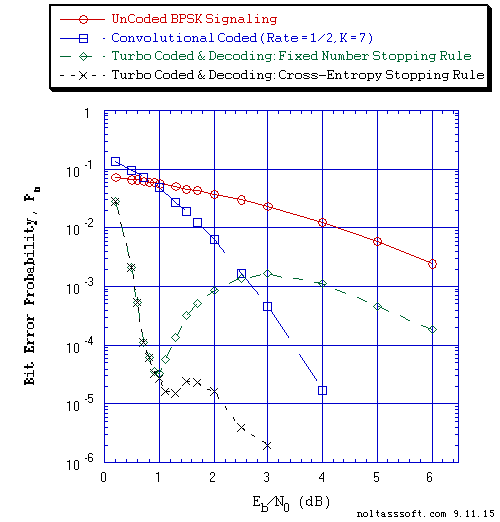```Figure 7. Bit Error Probability for UnCoded, Rate = 1/2 Convolutional Coded,
and Rate = 1/3 Turbo Coded (TC) BPSK Signaling over a Coherent
Memoryless Channel with Additive White Gaussian Noise (AWGN):
```
```          Equal probable Independent and Identical Distributed  (IID) Source
for 10 Million, 1 Million, and 1,001,472 Information Bits for
UnCoded, Convolutional Coded, and Turbo Coded BPSK Signaling
respectively over a Vector Channel;
```
```          Rate = 1/2, Constraint Length K = 7, (3,1,0,3,3,2,3), a Best
(Optimal) Non-Recursive Convolutional Code (J.P. Odenwalder) and
Viterbi Algorithm using a Path Memory Length of 35 bits and an
Unquantized Branch Metric;
```
```          Rate (r) = 1/3, K = 3 Turbo Code based on a r = 1/2, K = 3 (G0 = 7
octal, G1 = 5 octal) Recursive Systematic Convolutional component
code and a 6144-Bit QPP Turbo Encoder Interleaver; and
```
```          Log-MAP Iterative Turbo Decoder using Systematic Channel Output as
input to both component decoders and Decode Information Bit Decision
is based on the Sum of both decoders' log-likelihood ratios (L-values)
and for Fixed Number (6 Iterations) and Cross-Entropy (6 Iterations
Maximum) Stopping Rules.
```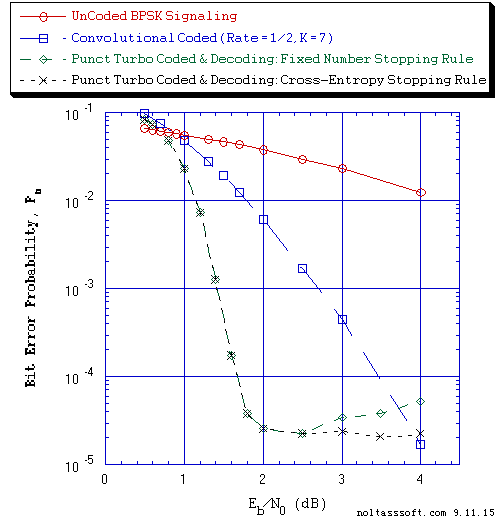```Figure 8. Bit Error Probability for UnCoded, Rate = 1/2 Convolutional Coded,
and Rate = 1/2 Punctured Turbo Coded (PTC) BPSK Signaling over a
Coherent Memoryless Channel with Additive White Gaussian Noise
(AWGN):
```
```          Equal probable Independent and Identical Distributed (IID) Source
for 10 Million, 1 Million, and 1,001,472 Information Bits for UnCoded,
Convolutional Coded and PTC BPSK Signaling respectively over a Vector
Channel;
```
```          Rate = 1/2, Constraint Length K = 7, (3,1,0,3,3,2,3), a Best
(Optimal)Non-Recursive Convolutional Code (J.P. Odenwalder) and
Viterbi Algorithm using a Path Memory Length of 35 bits and an
Unquantized Branch Metric;
```
```          Rate (r) = 1/5, K = 4 Parent Turbo Code based on a r = 1/3, K = 4
(G0 = 13 octal, G1 = 15 octal, G2 = 17 octal) Recursive Systematic
Convolutional component code (Optimum-weight Spectrum) and a
6144-Bit QPP Turbo Encoder Interleaver;
```
```          Punctured Turbo Code Rate = 1/2 for Puncturing Period of 2 and
Puncturing Matrix = [[1,1],[1,0],[0,0][0,1],[0,0]]; and
```
```          Log-MAP Iterative Turbo Decoder using Systematic Channel Output as
input to both component decoders and Decoder Information Bit Decision
is based on the Sum of both decoders' log-likelihood ratios (L-values)
and for Fixed Number (3 Iterations) and Cross-Entropy (3 Iterations
Maximum) Stopping Rules.
```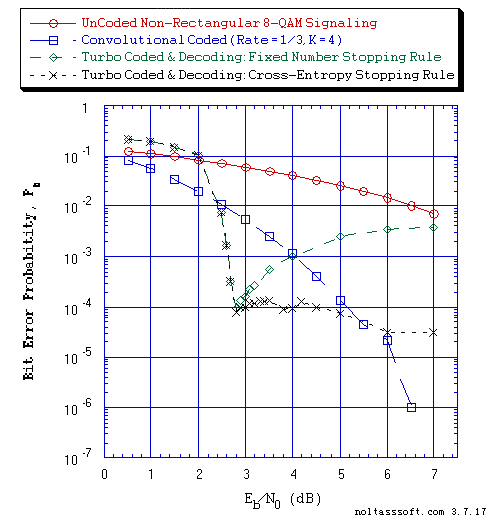```Figure 9. Bit Error Probability for UnCoded, Rate = 1/3 Convolutional Coded,
and Rate = 1/3 Turbo Coded (TC) Non-Rectangular 8-QAM Signaling over a
Coherent Memoryless Channel with Additive White Gaussian Noise (AWGN):
```
```          Equal probable Independent and Identical Distributed (IID) Source
for 10,000,002, 1 Million, and 1,001,472 Information Bits for
UnCoded, Convolutional Coded, and Turbo Coded Non-Rectangular (NR)
8-QAM Signaling respectively over a Vector Channel;
```
```          Rate = 1/3, Constraint Length K = 4, (7,5,6,7), a Best (Optimal)
Non-Recursive Convolutional Code (J.P. Odenwalder) and Viterbi
Algorithm using a Path Memory Length of 20 bits and an Unquantized
Branch Metric;
```
```          Rate (r) = 1/3, K = 4 Turbo Code based on a r = 1/2, K = 4
(G0 = 13 octal, G1 = 17 octal) Recursive Systematic Convolutional
component code (Optimum-weight Spectrum) and a 6144-Bit QPP Turbo
Encoder Interleaver;
```
```          NR 8-QAM Demodulation Constellation DeMapper Algorithm: Max-Log Bit
Metrics; and
```
```          Max-Log-MAP Iterative Turbo Decoder using Systematic Channel Output
as input to both component decoders and Decode Information Bit Decision
is based on the Sum of both decoders' log-likelihood ratios (L-values)
and for Fixed Number (5 Iterations) and Cross-Entropy (5 Iterations
Maximum) Stopping Rules.
```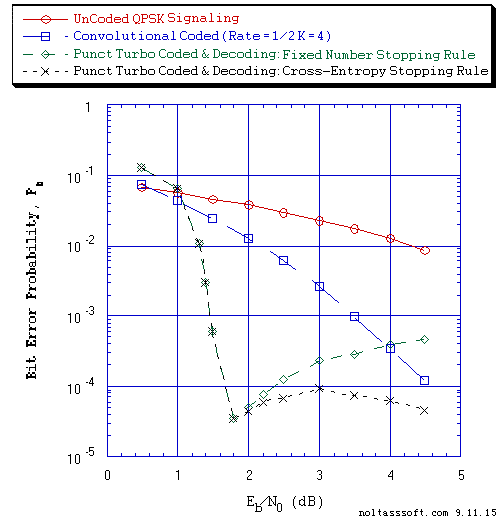```Figure 10. Bit Error Probability for UnCoded, Rate = 1/2 Convolutional Coded,
and Rate = 1/2 Punctured Turbo Coded (PTC) Gray Coded QPSK Signaling
over a Coherent Memoryless Channel with Additive White Gaussian Noise
(AWGN):
```
```          Equal probable Independent and Identical Distributed (IID)
Source for 10 Million, 1 Million, and 1,001,472 Information Bits for
UnCoded, Convolutional Coded, and PTC Gray Coded (GC) QPSK Signaling
respectively over a Vector Channel;
```
```          Rate = 1/2, Constraint Length K = 4, (3,2,3,3), a Best (Optimal)
Non-Recursive Convolutional Code (J.P. Odenwalder) and Viterbi
Algorithm using a Path Memory Length of 20 bits and an Unquantized
Branch Metric;
```
```          Rate (r) = 1/3, K = 4 Parent Turbo Code based on a r = 1/2, K = 4
(G0 = 13 octal, G1 = 17 octal) Recursive Systematic Convolutional
component code (Optimum-weight Spectrum) and a 6144-Bit QPP Turbo
Encoder Interleaver;
```
```          Punctured Turbo Code Rate = 1/2 for Puncturing Period of 2 and
Puncturing Matrix = [[1,1],[1,0],[0,1]];
```
```          GC QPSK Demodulation Constellation DeMapper Algorithm: Max-Log
Bit Metrics; and
```
```          Max-Log-MAP Iterative Turbo Decoder using Systematic Channel Output
as input to both component decoders and Decoder Information Bit
Decision is based on the Sum of both decoders' log-likelihood ratios
(L-values) and for Fixed Number (4 Iterations) and Cross-Entropy
(4 Iterations Maximum) Stopping Rules.
```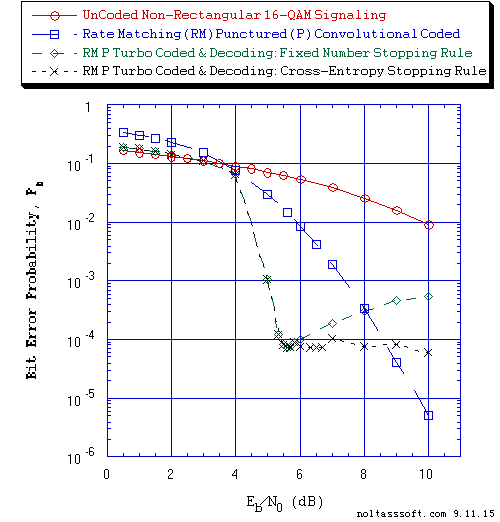```Figure 11. Bit Error Probability for UnCoded, Rate Matching (RM) Punctured
Convolutional Coded (PCC), and RM Punctured Turbo Coded PTC)
'Impure' Gray Coded Non-Rectangular (NR) 16-QAM Signaling over a
Coherent Memoryless Channel with Additive White Gaussian Noise (AWGN):
```
```          Equal probable Independent and Identical Distributed (IID)
Source for 10 Million, 1 Million, and 1,001,472 Information Bits
for UnCoded, RM PCC, and RM PTC Gray Coded NR 16-QAM Signaling
respectively over a Vector Channel;
```
```          Parent Code Rate = 1/3, K = 4, (7,5,6,7) a Non-Recursive
Convolutional Code and Viterbi Algorithm Decoder using a Path Memory
Length of 48 bits and a squared Euclidean distance Branch Metric;
```
```          Punctured Code (Rp = 2/4) derived from this 1/3 rate parent code
for Puncturing Period of 2 using the Puncturing Matrix
P = [[1,0],[1,1],[0,1]];
```
```          Rate (r) = 1/3, K = 4 Parent Turbo Code based on a r = 1/2, K = 4
(G0 = 13 octal, G1 = 17 octal) Recursive Systematic Convolutional
component code (Optimum-weight Spectrum) and a 6144-Bit QPP Turbo
Encoder Interleaver;
```
```          Punctured Turbo Code Rate = 1/2 for Puncturing Period of 2 and
Puncturing Matrix P = [[1,1],[1,0],[0,1]];
```
```          'Impure' Gray Code NR 16-QAM Demodulation Constellation DeMapper
Algorithm: Max-Log Bit Metrics; and
```
```          Max-Log-MAP Iterative Turbo Decoder using Systematic Channel Output
as input to both component decoders and Decoder Information Bit
Decision is based on the Sum of both decoders' log-likelihood ratios
(L-values) and for Fixed Number (4 Iterations) and Cross-Entropy
(4 Iterations Maximum) Stopping Rules.
```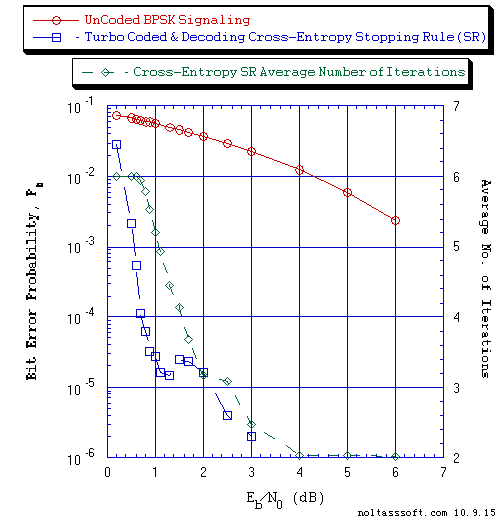```Figure 12. Bit Error Probability for UnCoded and Rate = 1/3 Turbo Coded (TC)
BPSK Signaling over a Coherent Memoryless Channel with Additive White
Gaussian Noise (AWGN):
```
```          Equal probable Independent and Identical Distributed  (IID) Source
for 10 Million and 1,001,472 Information Bits for UnCoded and
Turbo Coded BPSK Signaling over a Vector Channel, respectively;
```
```          Rate (r) = 1/3, K = 3 Turbo Code based on a r = 1/2, K = 3 (G0 = 7
octal, G1 = 5 octal) Recursive Systematic Convolutional component
code and a 6144-Bit QPP Turbo Encoder Interleaver; and
```
```          Log-MAP Iterative Turbo Decoder using Systematic Channel Output as
input to both component decoders and Decode Information Bit Decision
is based on the Sum of both decoders' log-likelihood ratios (L-values)
and for Cross-Entropy (6 Iterations Maximum) Stopping Rule.
```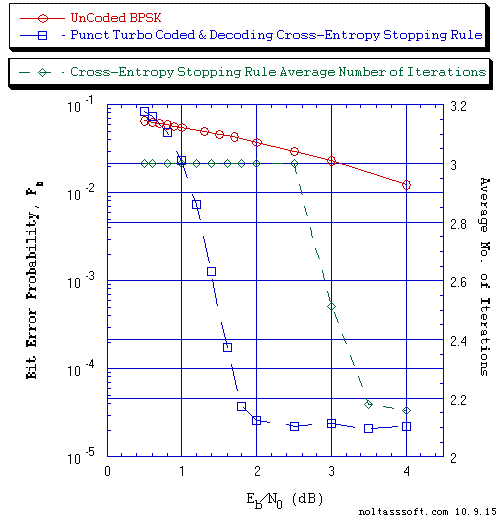```Figure 13. Bit Error Probability for UnCoded and Rate = 1/2 Punctured Turbo
Coded (PTC) BPSK Signaling over a Coherent Memoryless Channel
with Additive White Gaussian Noise (AWGN):
```
```          Equal probable Independent and Identical Distributed (IID) Source
for 10 Million and 1,001,472 Information Bits for UnCoded and PTC BPSK
Signaling over a Vector Channel, respectively;
```
```          Rate (r) = 1/5, K = 4 Parent Turbo Code based on a r = 1/3, K = 4
(G0 = 13 octal, G1 = 15 octal, G2 = 17 octal) Recursive Systematic
Convolutional component code (Optimum-weight Spectrum) and a
6144-Bit QPP Turbo Encoder Interleaver;
```
```          Punctured Turbo Code Rate = 1/2 for Puncturing Period of 2 and
Puncturing Matrix = [[1,1],[1,0],[0,0][0,1],[0,0]]; and
```
```          Log-MAP Iterative Turbo Decoder using Systematic Channel Output as
input to both component decoders and Decoder Information Bit Decision
is based on the Sum of both decoders' log-likelihood ratios (L-values)
and Cross-Entropy (3 Iterations Maximum) Stopping Rule.
```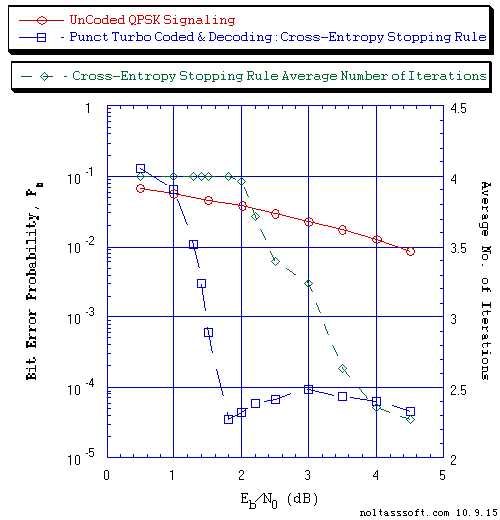```Figure 14. Bit Error Probability for UnCoded and Rate = 1/2 Punctured Turbo
Coded (PTC) Gray Coded QPSK Signaling over a Coherent Memoryless
Channel with Additive White Gaussian Noise (AWGN):
```
```          Equal probable Independent and Identical Distributed (IID) Source
for 10 Million and 1,001,472 Information Bits for UnCoded and PTC
Gray Coded (GC) QPSK Signaling over a Vector Channel, respectively;
```
```          Rate (r) = 1/3, K = 4 Parent Turbo Code based on a r = 1/2, K = 4
(G0 = 13 octal, G1 = 17 octal) Recursive Systematic Convolutional
component code (Optimum-weight Spectrum) and a 6144-Bit QPP Turbo
Encoder Interleaver;
```
```          Punctured Turbo Code Rate = 1/2 for Puncturing Period of 2 and
Puncturing Matrix = [[1,1],[1,0],[0,1]];
```
```          GC QPSK Demodulation Constellation DeMapper Algorithm: Max-Log
Bit Metrics; and
```
```          Max-Log-MAP Iterative Turbo Decoder using Systematic Channel Output
as input to both component decoders and Decoder Information Bit
Decision is based on the Sum of both decoders' log-likelihood ratios
(L-values) and for Cross-Entropy (4 Iterations Maximum) Stopping Rule.
```